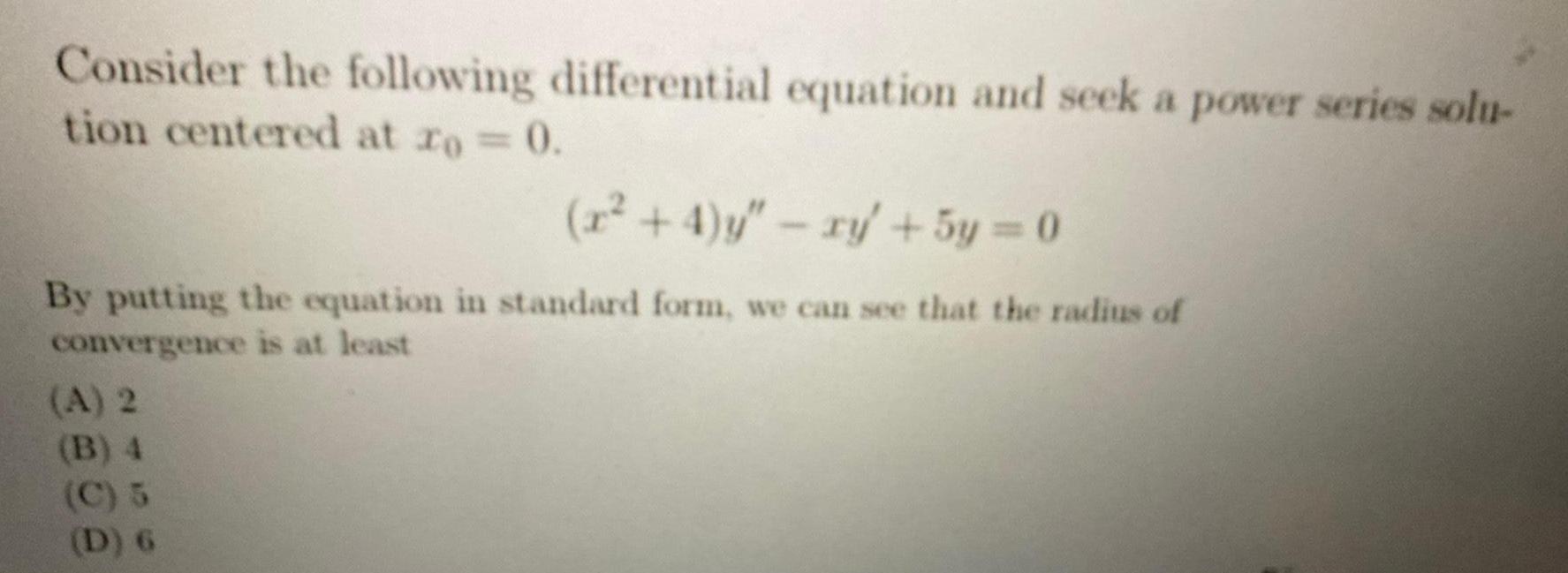Question:

# Consider the following differential equation and seek a

Last updated: 8/14/2022Consider the following differential equation and seek a power series solu- tion centered at ro = 0. (x²+4)y" - xy + 5y = 0 By putting the equation in standard form, we can see that the radius of convergence is at least (A) 2 (B) 4 (C) 5 (D) 6